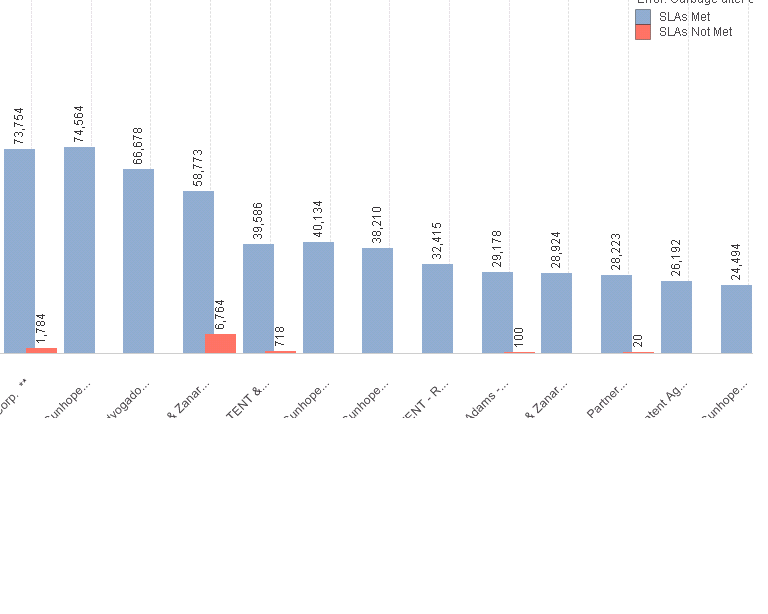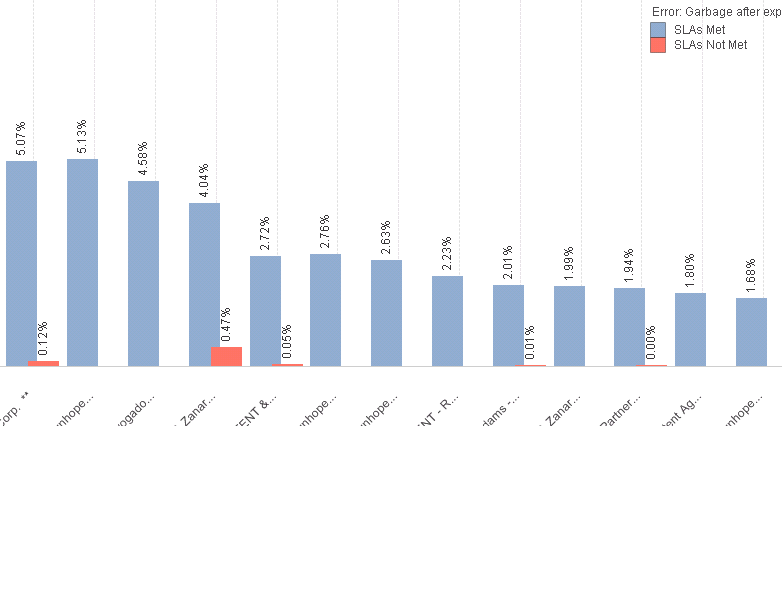# QlikView App Development

Discussion Board for collaboration related to QlikView App Development.

Honored Contributor II

## percentage calculation

hi,

my dimensions are

1.Name

2. calculated dimension is "=If(Networkdays(Scheduled_Send_Time__c,Delivery_Acknowledged_Time__c,\$(ListOfHolydays))>=6,'SLAs Not Met','SLAs Met')"

and expression is--> =Count(IP_No__c) ,So I'm getting the below screen results.Expected values/output are: Instituted of numbers I need percentages for example first dimension in above chart shows "73,754 and 1784" for the same expected out is "97.63% and 2.36%"

any help?

thanks

1 Solution

Accepted SolutionsMVP

## Re: percentage calculation

Qualify the total with Name, like this

Count(IP_No__c) / count(Total <Name> IP_No__c)

Logic will get you from a to b. Imagination will take you everywhere. - A Einstein
10 RepliesMVP

## Re: percentage calculation

Have you tried selecting relative?Partner

## Re: percentage calculation

Count(IP_No__c) / count(Total IP_No__c)

I can walk on water when it freezesMVP & Luminary

## Re: percentage calculation

HI,

Try like this

=Count(IP_No__c) / Count(TOTAL IP_No__c)

Or select relative option.

Regards,

Jagan.

Honored Contributor II

## Re: percentage calculation

hi Sunny/Jagan,

i tried both by applying Relative or ount(IP_No__c) / Count(TOTAL IP_No__c) geting the belwo result.the problem with above chart is the percentages are calculating with respect to all the dimensions("corp,nphoe....,ent AG,mhope" equalizes to 100%),my case each dimension is equalizes to 100%(for example the first dimension corp is one dimension combination of sla met & not met)

any help?

thanksMVP

## Re: percentage calculation

Qualify the total with Name, like this

Count(IP_No__c) / count(Total <Name> IP_No__c)

Logic will get you from a to b. Imagination will take you everywhere. - A Einstein
Honored Contributor III

## Re: percentage calculation

Your legend is showing some error, could you see why its showing error? You didn't mention in problem so I thought may be missed in checking that.

Honored Contributor II

## Re: percentage calculation

Thanks Jonathan.

Thank you allMVP

## Re: percentage calculation

if you want the total according to one of the dimension values ...then use the TOTAL key word along with the expression

like count(ID)/count(distinct TOTAL<dimension1>ID)

Honored Contributor II

## Re: percentage calculation

hi guys,

i 've to include avg line by considering only sla met cases.

can help some one?

thanks